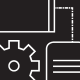# [NoBrainer] Automating Sparx Enterprise Architect with PowerShell

It has been some time since I have last posted something on our blog, so I will start with something easy: today I made the switch from Sparx Enterprise Architect v13.5 to v14.0. And yes, it really paid off since I now can actually decipher all those small connector icons on my Surface Book 2 screen which has a resolution of more than 3000px horizontally …

While I was at it I needed to extract a couple of diagrams from a model so I decided to give PowerShell a try and have it automated for me. As it turned out it was quite easy (except for a few things – more on that later).

In EA it all starts with the `Object Model` which shows you roughly how to get started in `C#`, `Java` and some script languages. EA being exposed as an ActiveX COM object for `PowerShell` loading a repository looks like this:

```# preparing the scene
cd "C:\Program Files (x86)\Sparx Systems\EA";
Add-Type -Path 'C:\Program Files (x86)\Sparx Systems\EA\Interop.EA.dll';

# instantiating the COM object
# NOTE: this will not work reliably
# \$ea = [EA.RepositoryClass]::new();
\$ea = New-Object -ComObject EA.Repository

\$modelPath = 'C:\MyFolder';
\$modelPathAndFileName = Join-Path -Path \$modelPath -ChildPath 'MyModel.eap';
\$result = \$ea.OpenFile(\$modelPathAndFileName);
#\$result must be \$true
```

A `repository` is also a COM object, so inspecting types is a little bit harder as usual, as all you see is: `__ComObject`. Each `repository` has a collection of `Models` which in turn contain a `Packages` collection. In each `Package` you find a `Diagrams` collection which may hold zero or more diagrams.

Exporting a `diagram` is limited to `PDF`, `PNG`, `JPG` and `EMF` via `\$diagram.SaveAsPDF()` and `\$diagram.SaveImagePage()`. The documentation is sparse but enough to start. A speciality with images is that you have to export an image for each page of the diagram whereas with PDF the whole diagram is exported.
This means you have to check on every diagram of how many pages it consists and save them with separate calls:

```for(\$pageWidth = 1; \$pageWidth -le \$diagram.PageWidth; \$pageWidth++)
{
for(\$pageHeight = 1; \$pageHeight -le \$diagram.PageHeight; \$pageHeight++)
{
\$diagramImgPathAndFileName = Join-Path -Path \$modelPath -ChildPath ('img\{0}-{1}-x{2}-y{3}.png' -f \$diagram.Name, \$diagram.DiagramGUID, \$pageWidth, \$pageHeight);
\$result = \$diagram.SaveImagePage(\$pageWidth, \$pageHeight, 0, 0, \$diagramImgPathAndFileName, 0)
}
}
```

In order to extract all diagrams in a repository we can crawl the package tree recursively as shown in the following script:

```# preparing the scene
cd "C:\Program Files (x86)\Sparx Systems\EA";
Add-Type -Path 'C:\Program Files (x86)\Sparx Systems\EA\Interop.EA.dll';

# instantiating the COM object
# NOTE: this will not work reliably
# \$ea = [EA.RepositoryClass]::new();
\$ea = New-Object -ComObject EA.Repository

\$modelPath = 'C:\MyFolder';
\$modelPathAndFileName = Join-Path -Path \$modelPath -ChildPath 'MyModel.eap';
\$result = \$ea.OpenFile(\$modelPathAndFileName);
#\$result must be \$true

function Process-Packages(\$packages)
{
if(!\$packages) { throw [System.ArgumentNullException]::new('packages'); }
if(0 -ge \$packages.Count) { return; }

foreach(\$package in \$packages)
{
foreach(\$diagram in \$diagrams)
{
\$diagramePdfPathAndFileName = Join-Path -Path \$modelPath -ChildPath ('img\{0}-{1}.pdf' -f \$diagram.Name, \$diagram.DiagramGUID);
\$result = \$diagram.SaveAsPDF(\$diagramePdfPathAndFileName)

if(1 -ge \$diagram.PageWidth -and 1 -ge \$diagram.PageHeight)
{
\$diagramImgPathAndFileName = Join-Path -Path \$modelPath -ChildPath ('img\{0}-{1}.png' -f \$diagram.Name, \$diagram.DiagramGUID);
\$result = \$diagram.SaveImagePage(1, 1, 0, 0, \$diagramImgPathAndFileName, 0);
continue;
}

for(\$pageWidth = 1; \$pageWidth -le \$diagram.PageWidth; \$pageWidth++)
{
for(\$pageHeight = 1; \$pageHeight -le \$diagram.PageHeight; \$pageHeight++)
{
\$diagramImgPathAndFileName = Join-Path -Path \$modelPath -ChildPath ('img\{0}-{1}-x{2}-y{3}.png' -f \$diagram.Name, \$diagram.DiagramGUID, \$pageWidth, \$pageHeight);
\$result = \$diagram.SaveImagePage(\$pageWidth, \$pageHeight, 0, 0, \$diagramImgPathAndFileName, 0)
}
}
}
Process-Packages \$package.Packages;
}
}

function Get-EaPackages(\$package)
{
if(!\$package) { throw [System.ArgumentNullException]::new('package'); }

\$result = [System.Collections.ArrayList]::new();

foreach(\$item in \$package.Packages)
{
}

return \$result;
}

{
if(!\$package) { throw [System.ArgumentNullException]::new('package'); }

\$result = [System.Collections.ArrayList]::new();

foreach(\$item in \$package.Diagrams)
{
}

return \$result;
}

foreach(\$model in \$ea.models)
{
Process-Packages \$model.packages;
}

\$ea.CloseFile();
\$ea.Exit();
```

A downside while exporting images is, that every image has always the size of a full page – regardless its actual size, as you can see in the following image (notice all the white space at the bottom):

Note: see the comment at the end of the post on how this can be worked around.

# Summary

Automating EA is easy, but the API has a very weak documentation and lacks good samples. Methods are only partially documented and you have to try and error a lot. Doing this in C# does not make things easier as there you have to use the same COM interop library. At least automation is possible …

Make sure to close the file and call `Exit()` on the repository, otherwise you have leftover EA processes in your system.

1.Arie Quist says:

Thanks a lot for this useful example of enumerating all diagrams – just what I need.
About the “page-by-page” issue of exporting to images : this can be circumvented by using the PutDiagramImageToFile() function of the EA Project class:

\$eaProject = \$ea.GetProjectInterface()

and then instead of \$diagram.SaveImagePage use

\$result = \$eaProject.PutDiagramImageToFile(\$diagram.DiagramGUID, \$diagramImgPathAndFileName, 1)

•Ronald Rink says:

2.MR Hưng says: